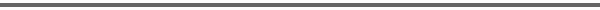Journal Home Page Cumulative Index List of all Volumes Complete Contentsof this Volume Previous Article Next Article Journal of Convex Analysis 11 (2004), No. 2, 391--400 Copyright Heldermann Verlag 2004On the Exact Value of Packing Spheres in a Class of Orlicz Function Spaces Ya Qiang Yan Dept. of Mathematics, Suzhou University, Suzhou, Jiangsu 215006, P. R. China, yanyq@pub.sz.jsinfo.net[Abstract-pdf] Main result: the packing constants of Orlicz function spaces $L^{(\Phi)}[0,1]$ and $L^{\Phi}[0,1]$ with Luxemburg and Orlicz norm have the exact value. \medskip (i) If $F_\Phi(t)=t\varphi(t)/\Phi(t)$ is decreasing, $1 2,$ then $$P(L^{(\Phi)}[0,1])=P(L^{\Phi}[0,1])=\frac{1}{1+2^{1/C_\Phi}},$$ where $C_\Phi=\lim\limits_{t\rightarrow\infty} F_\Phi(t)$. Keywords: Orlicz space, packing constants, Kottman constants. MSC 2000: 46E30. FullText-pdf (312 KB) for subscribers only.